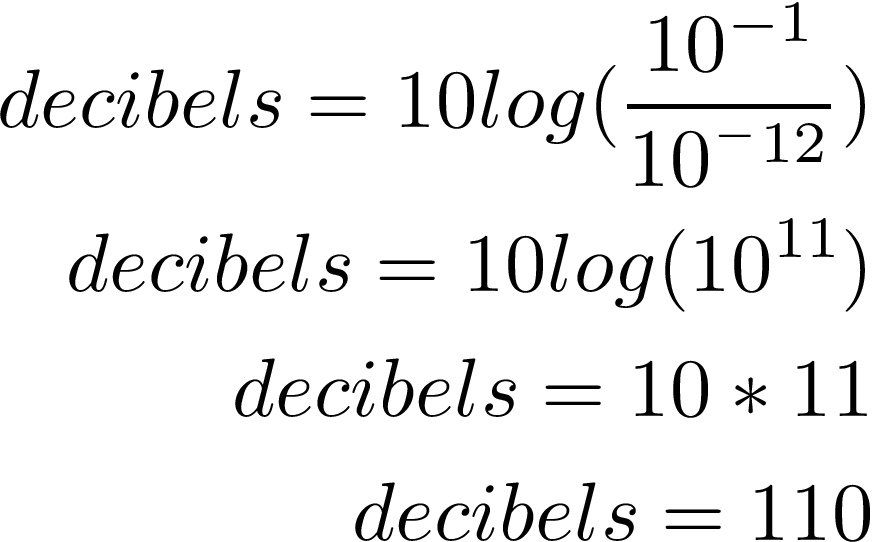# Logarithmic Models

A logarithm is the inverse of exponentiation. Depending on the situation one form is better than the other. This post will explore logarithms in greater detail.Converting Between Exponential and Logarithmic Form

There are times when it is necessary to convert an expression from exponential to logarithmic and vice versa. Below is an example of who the expression is rearranged form logarithm to exponential.The simplest way to explain I think is as follows

• for the logarithm, the exponent (y) and the base (a) are on opposite sides of the equal sign
• For the exponent form, the exponent (y) and base (a) are on the same side of the equal sign.

Here is an example using actual numbersAs you can see the exponent 3 and the base 2 are on opposite sides of the equal sign for the logarithmic form but er together for the exponential form.

When the base is e (Euler’s Number) it is known as a natural logarithmic function. e is the base rate growth of a continual process. The application of this is limitless. When the base is ten it is called a common logarithmic function.

Logarithmic Model Example

Below is an example of the application of logarithmic models

Exposure to noise above 120 dB can cause immediate pain and damage long-term exposure can lead to hearing loss. What aris the decimal level of a tv with an intensity of 10^1 watts per square inch.

First, we need the equation for calculating the decibel level.Now we plug in the information into the word problem for I and solveOur tv is dangerously loud and should include a warning message.  We dropped the negative sign because you cannot have negative decibel level.

Conclusion

Logarithms are another way to express exponential information and vice versa. It is the situation that determines which to use and the process of concert an expression from one to another is rather simple. In terms of solving actual problems, it is a matter of plugging numbers into an equation and allowing the calculator to work that allows you to find the answer.